# RS Aggarwal Solutions for Class 8 Maths Chapter 15 - Quadrilaterals

RS Aggarwal Solutions For Class 8 Maths Chapter 15 Quadrilaterals are provided here. You can download the pdf of RS Aggarwal Solutions for Class 8 Maths Chapter 15 Quadrilaterals from the given links. Class 8 is an important phase of a student’s life. It is critical to thoroughly understand the concepts taught in Class 8 as they are continued in Class 9 and 10, and also create a foundation. Here we will learn about the quadrilaterals, types of quadrilaterals and properties of quadrilaterals.

RS Aggarwal Solutions helps students to get a good score in the examinations, while also providing extensive knowledge about the subject, as Class 8 is a critical stage in their academic career. Therefore, we at BYJU’S provide answers to all questions uniquely and briefly.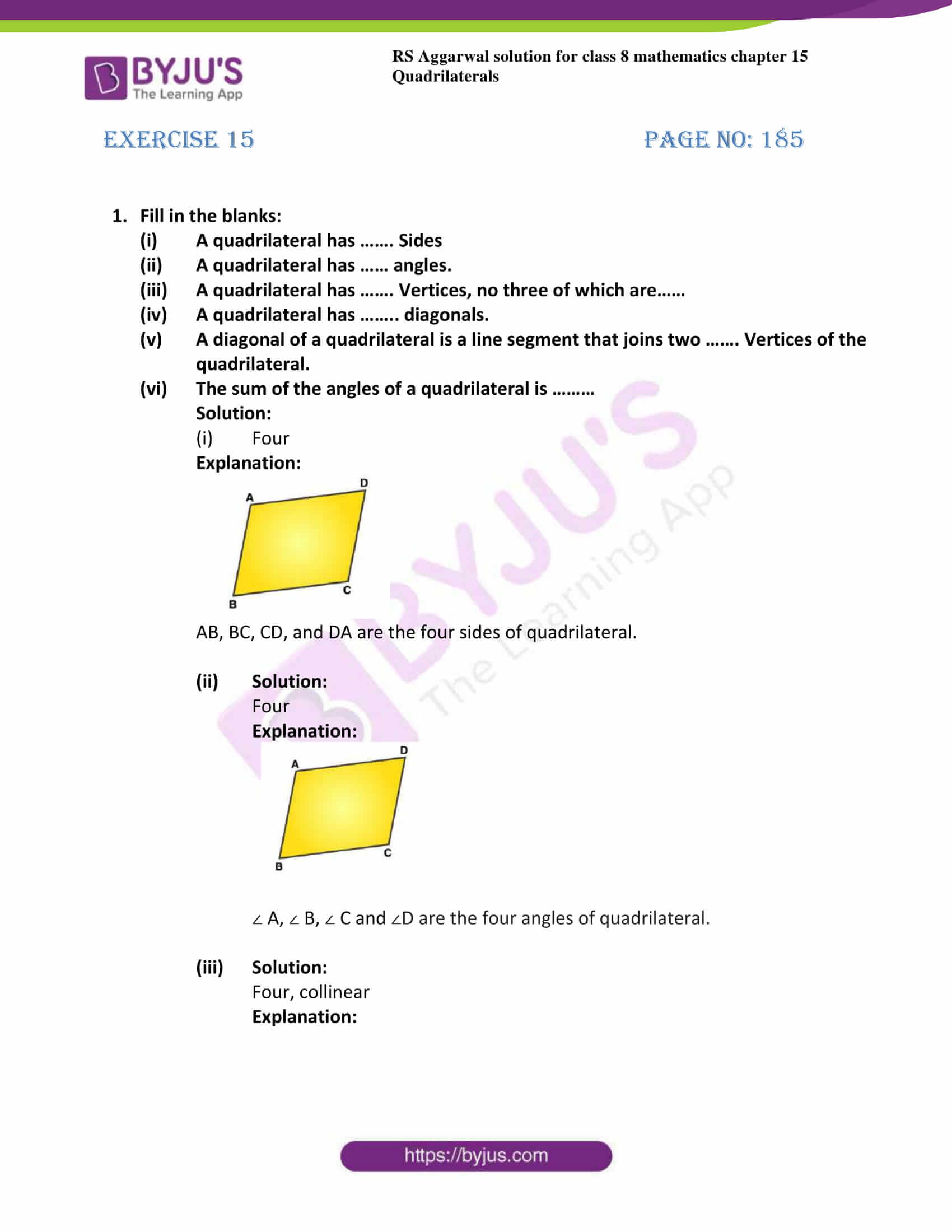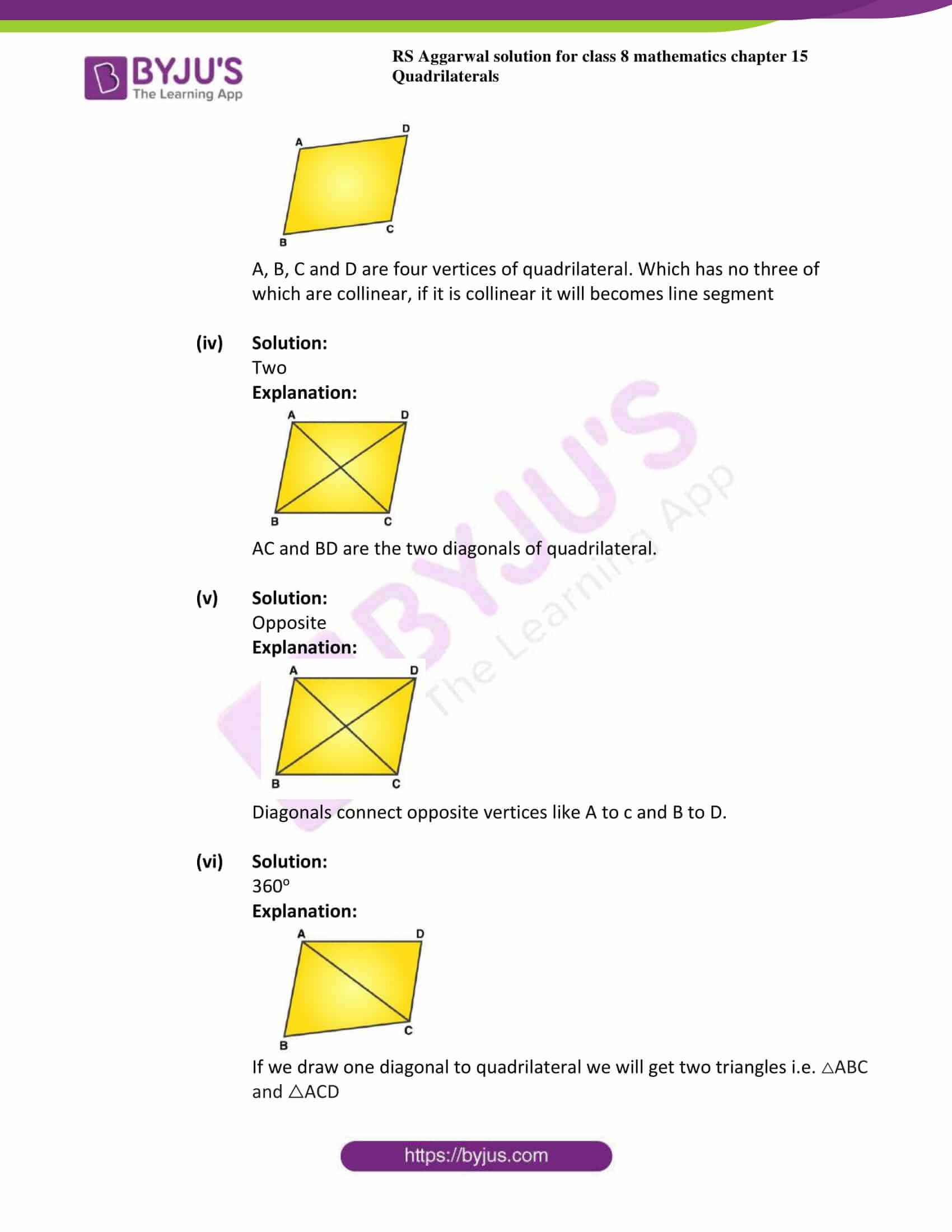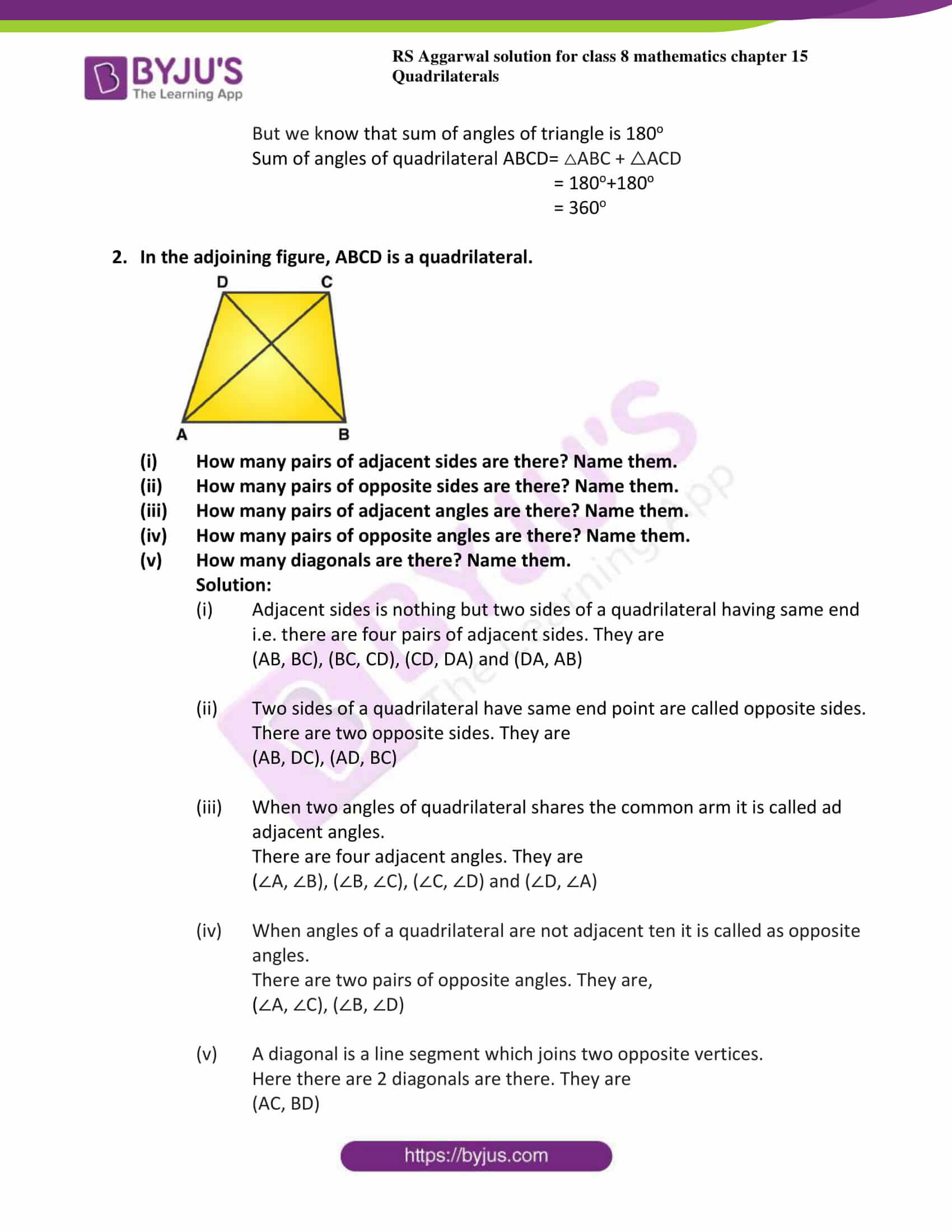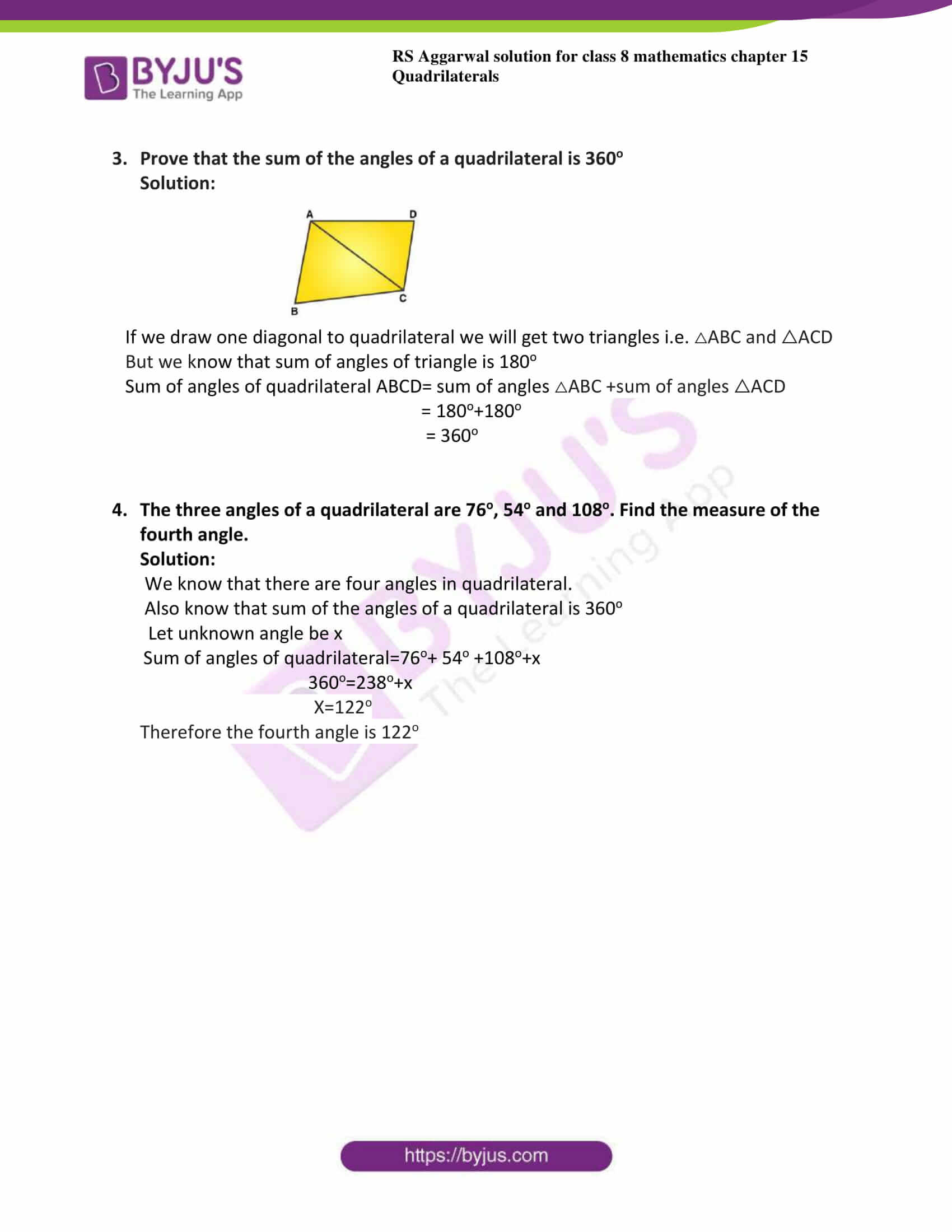## Exercise 15 Page

1. Fill in the blanks:

1. A quadrilateral has ……. Sides
2. A quadrilateral has …… angles.
3. A quadrilateral has ……. Vertices, no three of which are……
4. A quadrilateral has …….. diagonals.
5. A diagonal of a quadrilateral is a line segment that joins two ……. Vertices of the quadrilateral.
6. The sum of the angles of a quadrilateral is ………

Solution:

1. Four

Explanation: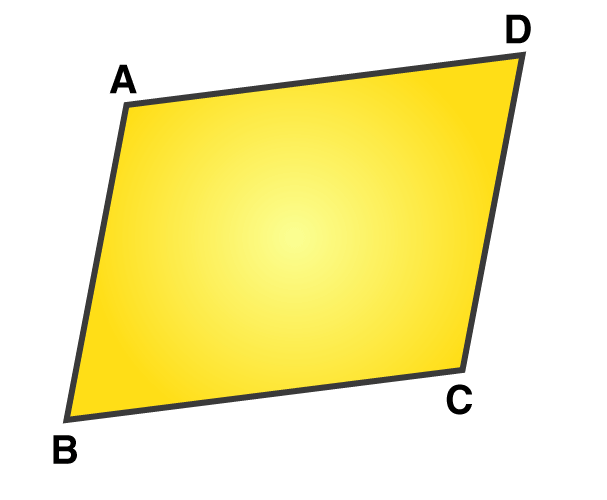AB, BC, CD, and DA are the four sides of quadrilateral.

1. Solution:

Four

Explanation:∠ A, ∠ B, ∠ C and ∠D are the four angles of quadrilateral.

1. Solution:

Four, collinear

Explanation:A, B, C and D are four vertices of quadrilateral. Which has no three of which are collinear, if it is collinear it will becomes line segment

1. Solution:

Two

Explanation: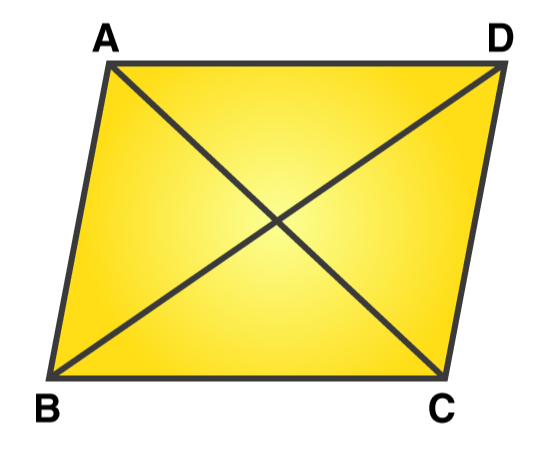AC and BD are the two diagonals of quadrilateral.

1. Solution:

Opposite

Explanation:Diagonals connect opposite vertices like A to c and B to D.

1. Solution:

360o

Explanation: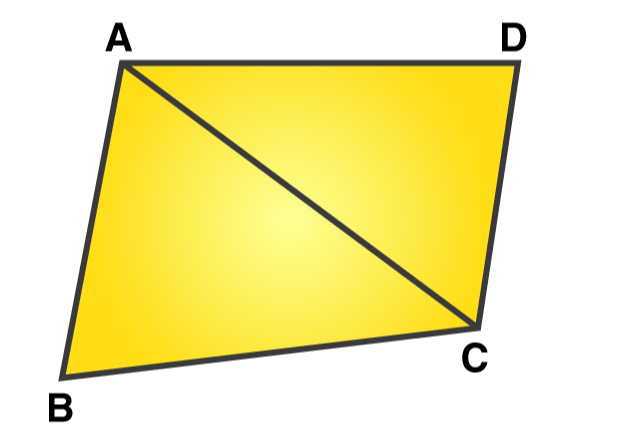If we draw one diagonal to quadrilateral we will get two triangles i.e. △ABC and △ACD

But we know that sum of angles of triangle is 180o

Sum of angles of quadrilateral ABCD= △ABC + △ACD

= 180o+180o

= 360o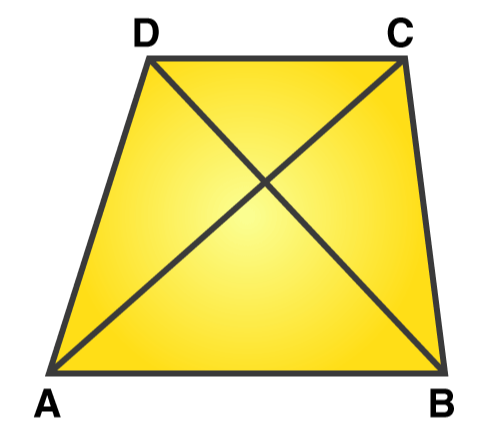1. How many pairs of adjacent sides are there? Name them.
2. How many pairs of opposite sides are there? Name them.
3. How many pairs of adjacent angles are there? Name them.
4. How many pairs of opposite angles are there? Name them.
5. How many diagonals are there? Name them.

Solution:

1. Adjacent sides is nothing but two sides of a quadrilateral having same end

i.e. there are four pairs of adjacent sides. They are

(AB, BC), (BC, CD), (CD, DA) and (DA, AB)

1. Two sides of a quadrilateral have same end point are called opposite sides.

There are two opposite sides. They are

There are four adjacent angles. They are

(∠A, ∠B), (∠B, ∠C), (∠C, ∠D) and (∠D, ∠A)

1. When angles of a quadrilateral are not adjacent ten it is called as opposite angles.

There are two pairs of opposite angles. They are,

(∠A, ∠C), (∠B, ∠D)

1. A diagonal is a line segment which joins two opposite vertices.

Here there are 2 diagonals are there. They are

(AC, BD)

3. Prove that the sum of the angles of a quadrilateral is 360o

Solution: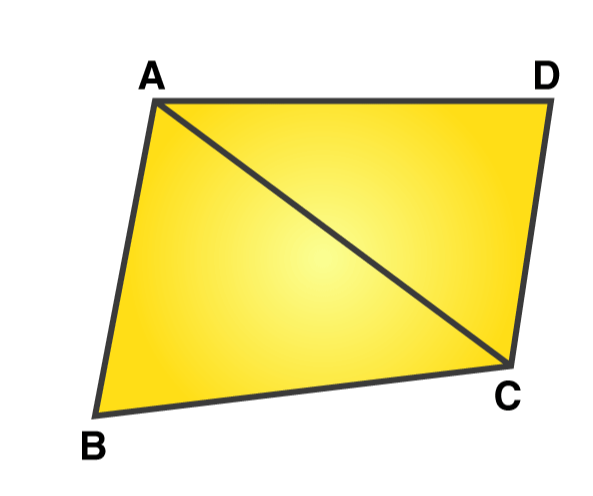If we draw one diagonal to quadrilateral we will get two triangles i.e. △ABC and △ACD

But we know that sum of angles of triangle is 180o

Sum of angles of quadrilateral ABCD= sum of angles △ABC +sum of angles △ACD

= 180o+180o

= 360o

4. The three angles of a quadrilateral are 76o, 54o and 108o. Find the measure of the fourth angle.

Solution:

We know that there are four angles in quadrilateral.

Also know that sum of the angles of a quadrilateral is 360o

Let unknown angle be x

Sum of angles of quadrilateral=76o+ 54o +108o+x

360o=238o+x

X=122o

Therefore the fourth angle is 122o

## RS Aggarwal Solutions for Class 8 Maths Chapter 15- Quadrilaterals

Chapter 15, Quadrilaterals, contains one Exercise. RS Aggarwal Solutions given here contains the answers to all the questions present in this exercise. Let us have a look at some of the concepts that are being discussed in this chapter.